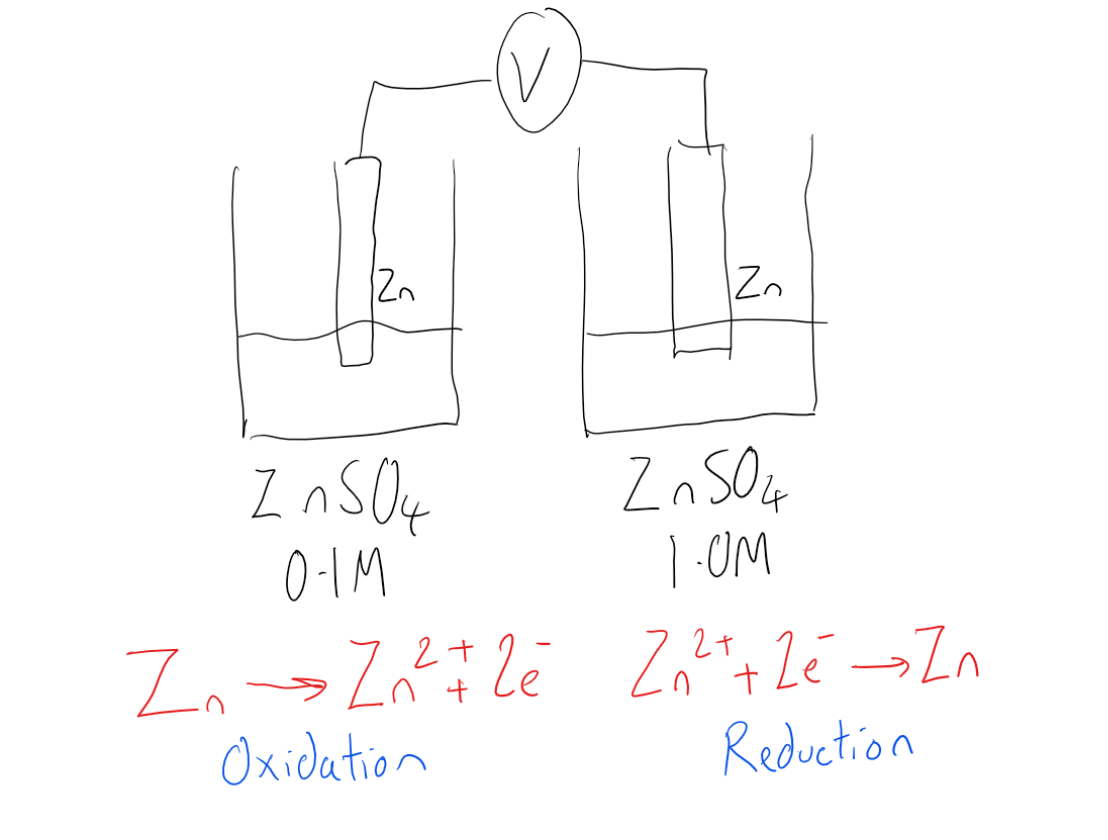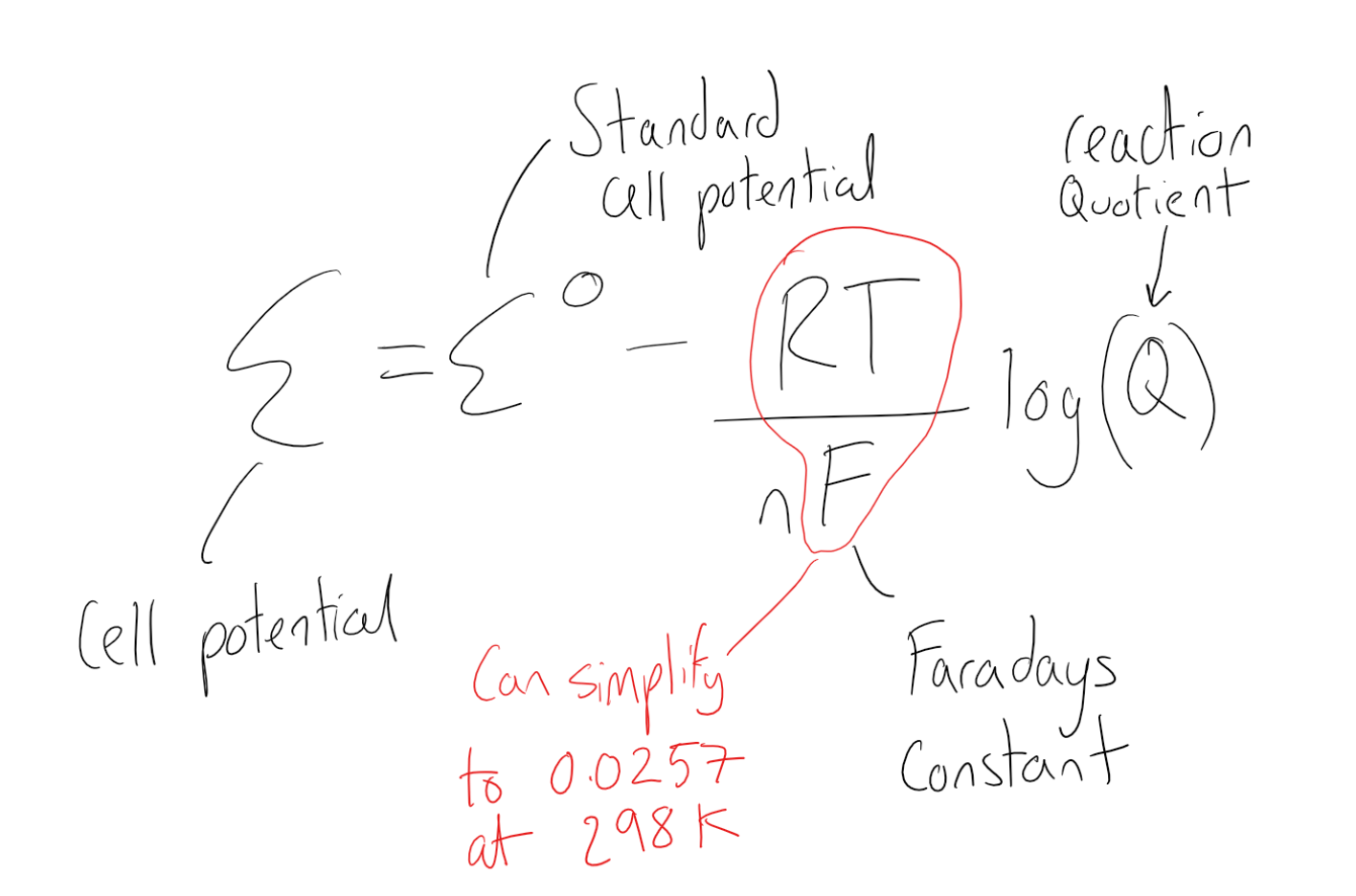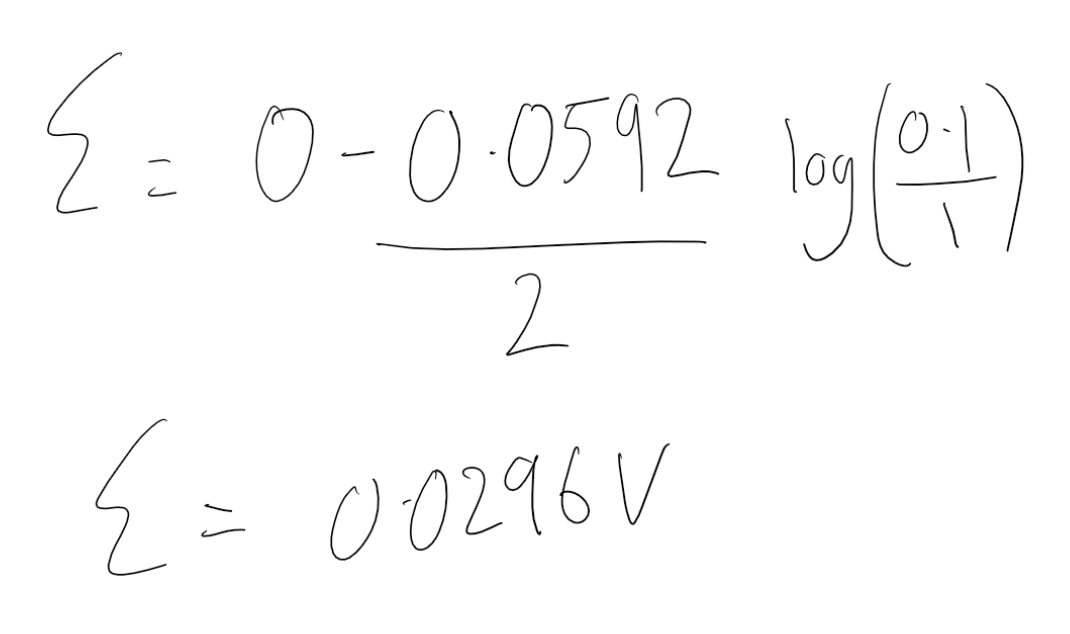Cyber Sale - Only 3 Days Remaining: ALL MCAT Products & Admissions Services

MCAT Content / Electrochemistry / Concentration Cell Direction Of Electron Flow Nernst Equation

### Concentration Cell: Direction of Electron Flow, Nernst Equation

Topic: Electrochemistry

In electrochemistry, the Nernst equation relates the reduction potential of an electrochemical reaction to the standard electrode potential, temperature, and activities of the chemical species undergoing reduction and oxidation.

The Nernst equation can be used, in conjunction with other information, to determine the reduction potential of a half-cell in an electrochemical cell. It can also be used to determine the total voltage, or electromotive force, for a full electrochemical cell.• E is the electromotive force of the non-standard cell
• Eo is the electromotive force of the standard cell
• n is the number of moles of electrons transferred in the reaction
• Q is the reaction quotient
• F is Faraday’s constant (96485 C mol-1)
• T is temperature (K)
• R is the gas constant (8.1345 J mol-1 K-1)

The standard cell potential can be calculated using reference values at standard temperatures and conditions. Q can be calculated by resolving the logarithm of the concentrations of the oxidized and reduced cell. RT and F can also be simplified if at 298K to 0.0257 to make the equation simpler.

A concentration cell is a voltaic cell that has the same electrodes but different concentrations of electrolytes on either side of the cell joined by a salt bridge. This difference in concentration is enough for a voltage to be generated.In the less concentrated cell, the electrode would break down to increase the concentration of ions in solution by oxidation. In the example Zn -> Zn2+ + 2e. The electrons move through the wire generating a voltage and end up at the more concentrated electrode. At the more concentrated electrode, the concentration of the ions in solution needs to be decreased. In the example the Zn2+ +2e -> Zn which is the zinc ions reducing itself and leaving solution. By canceling out the electrons and solid zinc we can see that the concentration moves from the 1.0M solution to the 0.1M solution.By using the Nernst equation we can calculate the cell potential. Using the values of concentrations in the concentration cell we can work out Q and from this using RT and the number of moles of electrons in this example 2 we can calculate the cell potential. The cell potential under standard conditions is 0 as the oxidation is +0.76v and the reduction is -0.76v.Practice Questions

Studying metabolism with galvanic cells

MCAT Official Prep (AAMC)

Key Points

• The Nernst equation can be used to determine the total voltage (electromotive force) for a full electrochemical cell.

• The Nernst equation gives a formula that relates the numerical values of the concentration gradient to the electric gradient that balances it.

• A concentration cell is a voltaic cell with differing concentrations but the same electrodes and electrolyte from which a cell potential can be calculated

Key Terms

Half-cell: Either of the two parts of an electrochemical cell containing an electrode and an electrolyte.

Electrode: The terminal through which electric current passes between metallic and nonmetallic parts of an electric circuit. In electrolysis, the electrodes are placed in the solution separately.

Voltaic cell: A cell, such as in a battery, in which an irreversible chemical reaction generates electricity; a cell that cannot be recharged.

Concentration cell: A galvanic cell in which the two half-cells are the same except for the concentration of the solutes; spontaneous when the overall reaction is the dilution of the solute

Faraday’s constant (F): The charge on 1 mol of electrons; F = 96,485 C/mol e−

Nernst equation: An equation that relates the logarithm of the reaction quotient (Q) to nonstandard cell potentials; can be used to relate equilibrium constants to standard cell potentials

Billing Information